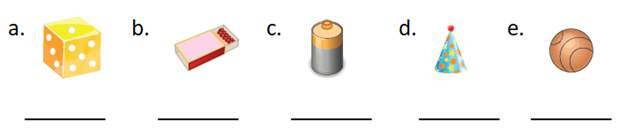Shapes Worksheet for Class 1

# Shapes Worksheet for Class 1

## Shapes Worksheet for Class 1

Shapes worksheets for class 1 are prepared by experts to clarify the concepts of Shapes class 1. After studying the concepts of Shapes class 1, revision of Shapes worksheets for class 1 is very important for the students. This assesses the preparation of the students. Students can assess themselves by solving the Shapes worksheet for class 1. Parents and teachers can also assess the students by giving them these Shapes worksheets for class 1 to solve.

Maths-formula brings such worksheets on Shapes Class 1 for you. By solving these Shapes worksheets for class 1, you can increase the understanding of the concepts learnt. These worksheets are prepared by experienced teachers and subject matter experts.

Unit tests can also be prepared by the teachers using Shapes worksheet for class 1.

## Shapes Worksheet for Class 1

1. Write horizontal line, vertical line or slanting line for each of the given lines.

2. Write curved line or straight line for each of the given lines.

3. Name each of the following solid shapes.

4. Write the shape of the following objects.

5. Name each of the following 2D shapes.

6. Write the shape of the following objects.

7. Write the number of edges, the number of faces and the number of vertices in each of the following solid shapes.

8. Write the number of sides and the number of corners in each of the following 2D shapes.

9. Write R for the objects which can roll, S for the objects which can slide and RS for the objects which can roll and slide both.10. Colour the triangles green, the squares red, the rectangles blue and the circles yellow. Count and write the number of triangles, the number of squares, the number of rectangles and the number of circles.

Number of triangles = ________

Number of squares = ________

Number of rectangles = ________

Number of circles = ________

11. Write the shape of the following objects in three dimensions.

a. A ludo coin _____________

b. A flute ___________________

c. A drum _________________

d. A match box _____________

e. An almirah _______________

f. A joker cap ________________

g. Rubik’s cube _____________

h. A ball __________________

i. A globe _________________

j. A pylon _________________

12. Write the shape of the following objects in two dimensions.

a. A LED TV screen ___________

b. Mobile screen _______________

c. A carrom board   __________

d. A cloth hanger ____________

f. A car wheel _______________

g. A chess board _____________

h. A plate ___________________

1. a. Vertical line

b. Horizontal line

c. Slanting line

2. a. Curved line

b. Straight line

c. Curved line

d. Straight line

3. a. Cube       b. Cuboid

c. Cone         d. Cylinder

e. Sphere

4. a. Cube          b. Cuboid

c. Cylinder    d. Cone

e. Sphere

5. a. Triangle     b. Square

c. Rectangle    d. Circle

6. a. Triangle      b. Circle

c. Rectangle     d. Square

7.

 Number of edges Number of faces Number of vertices a. 12 6 8 b. 12 6 8 c. 1 2 1 d. 2 3 0 e. 0 1 0

8.

 Number of sides Number of corners a. 3 3 b. 4 4 c. 4 4 d. 1 0

9. a. S         b. S

c. RS          d. RS

e. R

10. Number of triangles = 3

Number of squares = 1

Number of rectangles = 11

Number of circles = 3

11. a. Cube       b. Cylinder

c. Cylinder   d. Cuboid

e. Cuboid      f. Cone

g. Cube         h. Sphere

i. Sphere        j. Cone

12. a. Rectangle    b. Rectangle

c. Square        d. Triangle

e. Triangle      f. Circle

g. Square        h. CircleMaths Worksheets for Other Chapters of Class 1Maths Worksheets for Other Classes

Maths Worksheets for Class 2

Maths Worksheets for Class 3

Maths Worksheets for Class 4

Maths Worksheets for Class 5

Maths Worksheets for Class 6

Maths Worksheets for Class 7

Maths Worksheets for Class 8

Maths Worksheets for Class 9